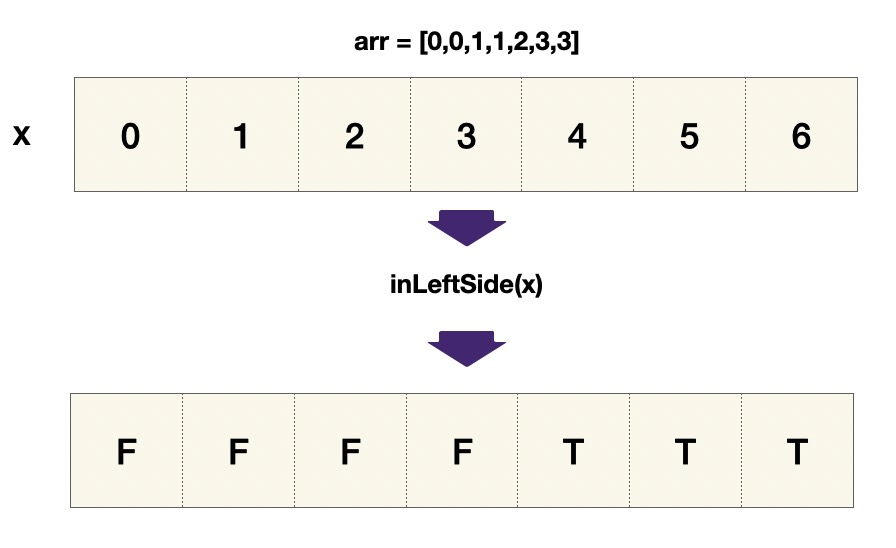# LeetCode Single Element in a Sorted Array Solution

You are given a sorted array consisting of only integers where every element appears exactly twice, except for one element which appears exactly once.

Return the single element that appears only once.

Your solution must run in `O(log n)` time and `O(1)` space.

Example 1:

Input: nums = [1,1,2,3,3,4,4,8,8] Output: 2

Example 2:

Input: nums = [3,3,7,7,10,11,11] Output: 10

Constraints:

• `1 <= nums.length <= 105`
• `0 <= nums[i] <= 105`

## Solution

Observe that the parity (even or odd) of indices ties closely with where the single element is. We know that the numbers come in pairs before and after the one single element `s`. For the pairs that is to the left of `s`: the first element takes an even index `e` (as array is 0 indexed) and the second element takes an odd index `e+1`. Then the single element `s` takes only one position (even), so that the pattern on the right of `s` is reversed. For the pairs that is to the right of `s`: the first element takes an odd index `o`, and the second element takes an even index `o+1`

Therefore, for an even index `e`, `nums[e]!=nums[e+1]` if the `s` is to the left of `o`. Similar for an odd index `o`, `nums[o]!=nums[o-1]` means `s` has already appeared. We must also keep an eye out for `out of bounds`, that is, to check whether `idx` is the last index in `nums`.#### Implementation

``````1def singleNonDuplicate(self, nums):
2    def to_the_left(idx):
3        if (idx == len(nums)-1):
4            return True
5        elif (idx % 2):   # odd
6            return nums[idx] != nums[idx-1]
7        else:             # even
8            return nums[idx] != nums[idx+1]
9
10    left, right, ans = 0, len(nums)-1, -1
11    while left <= right:
12        mid = (left + right) // 2
13        if to_the_left(mid):
14            ans = mid
15            right = mid - 1
16        else:
17            left = mid + 1
18
19    return nums[ans]  ``````